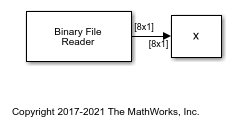# Change the Endianness of the Data in Simulink

By default, the Binary File Reader block uses the endianness of the host machine. To change the endianness, such as when the host machine that writes the data does not have the same endianness as the host machine that reads the data, use the `swapbytes` function.

Write a numeric array in big endian format into the file `bigEndian.dat`. Read the data using the `Binary File Reader` block. The reader reads the data in little endian format.

```fid = fopen('bigEndian.dat','w','b'); fwrite(fid,[1 2 3 4 5 6 7 8],'double'); fclose(fid); ```

Open and simulate the model.

```model = 'changeEndian'; open_system(model) sim(model) ```Display the data variable, x.

```display(x) ```
```x = 1.0e-318 * 0.3039 0.0003 0.0104 0.0206 0.0256 0.0307 0.0357 0.0408 ```

The array `x` does not match the original data. Change the endianness of `x` using the `swapbytes` function.

```y = swapbytes(x); display(y) ```
```y = 1 2 3 4 5 6 7 8 ```

That array `y` matches the original data.

## SupportGet trial now